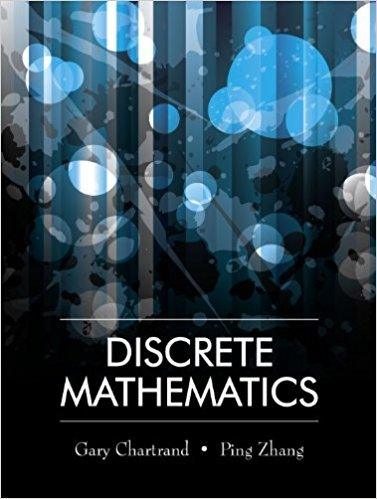×
×

# Solutions for Chapter 4: MATHEMATICAL INDUCTION## Full solutions for Discrete Mathematics | 1st Edition

ISBN: 9781577667308Solutions for Chapter 4: MATHEMATICAL INDUCTION

Solutions for Chapter 4
4 5 0 287 Reviews
31
2
##### ISBN: 9781577667308

Chapter 4: MATHEMATICAL INDUCTION includes 29 full step-by-step solutions. Discrete Mathematics was written by and is associated to the ISBN: 9781577667308. This textbook survival guide was created for the textbook: Discrete Mathematics, edition: 1. Since 29 problems in chapter 4: MATHEMATICAL INDUCTION have been answered, more than 10850 students have viewed full step-by-step solutions from this chapter. This expansive textbook survival guide covers the following chapters and their solutions.

Key Math Terms and definitions covered in this textbook
• Adjacency matrix of a graph.

Square matrix with aij = 1 when there is an edge from node i to node j; otherwise aij = O. A = AT when edges go both ways (undirected). Adjacency matrix of a graph. Square matrix with aij = 1 when there is an edge from node i to node j; otherwise aij = O. A = AT when edges go both ways (undirected).

• Affine transformation

Tv = Av + Vo = linear transformation plus shift.

• Associative Law (AB)C = A(BC).

Parentheses can be removed to leave ABC.

• Cofactor Cij.

Remove row i and column j; multiply the determinant by (-I)i + j •

• Commuting matrices AB = BA.

If diagonalizable, they share n eigenvectors.

• Companion matrix.

Put CI, ... ,Cn in row n and put n - 1 ones just above the main diagonal. Then det(A - AI) = ±(CI + c2A + C3A 2 + .•. + cnA n-l - An).

• Diagonalizable matrix A.

Must have n independent eigenvectors (in the columns of S; automatic with n different eigenvalues). Then S-I AS = A = eigenvalue matrix.

• Elimination.

A sequence of row operations that reduces A to an upper triangular U or to the reduced form R = rref(A). Then A = LU with multipliers eO in L, or P A = L U with row exchanges in P, or E A = R with an invertible E.

• Fibonacci numbers

0,1,1,2,3,5, ... satisfy Fn = Fn-l + Fn- 2 = (A7 -A~)I()q -A2). Growth rate Al = (1 + .J5) 12 is the largest eigenvalue of the Fibonacci matrix [ } A].

• Gram-Schmidt orthogonalization A = QR.

Independent columns in A, orthonormal columns in Q. Each column q j of Q is a combination of the first j columns of A (and conversely, so R is upper triangular). Convention: diag(R) > o.

• Least squares solution X.

The vector x that minimizes the error lie 112 solves AT Ax = ATb. Then e = b - Ax is orthogonal to all columns of A.

• Nilpotent matrix N.

Some power of N is the zero matrix, N k = o. The only eigenvalue is A = 0 (repeated n times). Examples: triangular matrices with zero diagonal.

• Orthonormal vectors q 1 , ... , q n·

Dot products are q T q j = 0 if i =1= j and q T q i = 1. The matrix Q with these orthonormal columns has Q T Q = I. If m = n then Q T = Q -1 and q 1 ' ... , q n is an orthonormal basis for Rn : every v = L (v T q j )q j •

• Pivot.

The diagonal entry (first nonzero) at the time when a row is used in elimination.

• Schwarz inequality

Iv·wl < IIvll IIwll.Then IvTAwl2 < (vT Av)(wT Aw) for pos def A.

• Solvable system Ax = b.

The right side b is in the column space of A.

• Trace of A

= sum of diagonal entries = sum of eigenvalues of A. Tr AB = Tr BA.

• Triangle inequality II u + v II < II u II + II v II.

For matrix norms II A + B II < II A II + II B II·

v + w = (VI + WI, ... , Vn + Wn ) = diagonal of parallelogram.

• Volume of box.

The rows (or the columns) of A generate a box with volume I det(A) I.

×